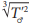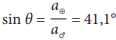# Practical Exercise 5: THE EARTH FROM MARS

Calculate the angular distance of the Earth from the Sun from the point of view of an observer on Mars. You know that the sidereal orbital period of Mars is 687 Earth days.

T ≐ 687days ≐ 1,88 years. We start from the 3rd Kepler’s law T ≐ 687days ≐ 1,88 years. We start from the 3rd Kepler’s law a' =≐ 1,52 au. From a similar geometry as in Figure 1 (only we calculate for Mars instead of the Earth) we get: T♂ ≐ 687days ≐ 1,88 years.
(only we calculate for Mars instead of the Earth) we get:.